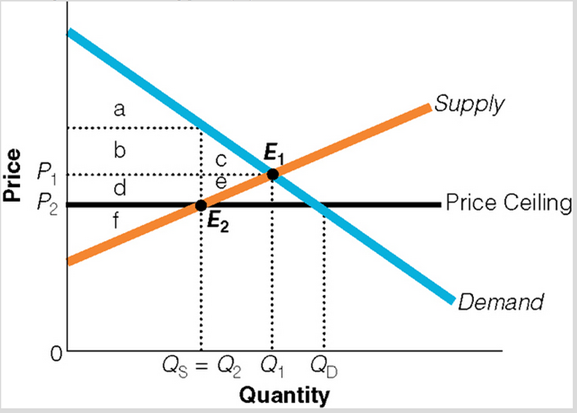### Create an Account

Home / Questions / Use the diagram to answer the following questions from (a-c). a)At the initial equilibri...

# Use the diagram to answer the following questions from (a-c). a)At the initial equilibrium price, which area represents consumer surplus? Which area represents the producer surplus? b)After the

Use the diagram to answer the following questions from (a-c).a)At the initial equilibrium price, which area represents consumer surplus? Which area represents the producer surplus?

b)After the price ceiling is imposed, which area represents consumer surplus? Which area represents the producer surplus?

c)Which area represents the deadweight loss cost of the price ceiling?

May 06 2021 View more View LessSubscribe To Get Solution